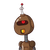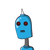# Rahim deposited 10000 rupees in a bank, which pays interest compounded half-yearly. The annual rate of interest is 10%. How

Rahim deposited 10000 rupees in a bank, which pays interest compounded half-
yearly. The annual rate of interest is 10%. How much should he get back after one
year?
Amount deposited, p =
Term n = one year=
half years
since the annual interest rate is 10%
therefore, interest rate for 6 months, r =
amount gets after one year =​

### 2 thoughts on “Rahim deposited 10000 rupees in a bank, which pays interest compounded half-<br />yearly. The annual rate of interest is 10%. How”

1.amound get after one year

2.Given : Rahim deposited 10000 rupees in a bank, which pays interest compounded half- yearly. The annual rate of interest is 10%. How much should he get back after one

year ?

we know that, When rate is compounded Half – Yearly :-

• Half yearly Means Half of a year, when we Multiply it with 2 we will get a year .

So we can say that in this case :-

• Rate is Divided by 2.
• Time is Multiply by 2 . ( To make it a complete year).

so,

• Rate = 10/2 = 5% per annum.
• Time = 1 * 2 = 2 years.

then,

→ Amount = P[1 + (R/100)]^time

→ A = 10000[1 + (5/100)]²

→ A = 10000[1 + (1/20)]²

→ A = 10000 * (21/20)²

→ A = (10000 * 441)/400

→ A = Rs.11025 (Ans.)

Hence, Rahim gets Rs.11025 back after one year .